WEBINAR
Magnetic Levitation for Energy Harvesting
Thursday, June 22, 2023
Time
SESSION 1
SESSION 2
CEST (GMT +2)
03:00 PM
08:00 PM
EDT (GMT -4)
09:00 AM
02:00 PM
HOME / Applications / Electric field inside the cavity of a charged sphere

# Electric field inside the cavity of a charged sphere

Used Tools:## Physics

Superposition principle states that if a single excitation is broken down into few constitutive components, total response is the sum of the responses to individual components. The use of the principle can be illustrated on the following electrostatic example.
Sphere of radiuswith an empty, spherical cavity of a radius, has a positive volume charge densityThe center of the cavity is at the distancefrom the center of the charged sphere (Figure 1).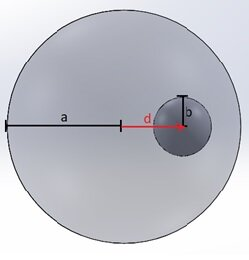Figure 1 - Positively charged sphere with an off-centered cavity

According to the superposition principle, total field inside the cavity can be found by adding up individual fields of:

• A positively charged (), thoroughly filled sphere with a radius.
• A negatively charged () sphere whose size and position match the cavity (Fig. 2).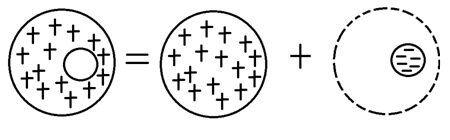Figure 2 - Representation of an empty volume by a superposition of two opposite charge density domains

Field of any isolated, uniformly charged sphere in its interior at a distance r, can be calculated from Gauss’ Law:Which yields for a positive sphere:And for a negative sphere:Where vectorsandare as defined in Figure 3.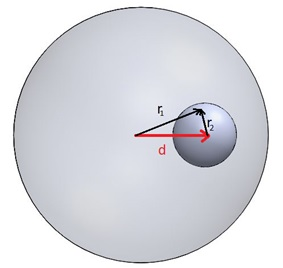Figure 3 - Relationship between the individual Electric field directions and the vector  representing the cavity offset

Therefore, the total electric field in the cavity can be computed as:From the last equation, it can be concluded that the electric field in the cavity is constant with a directionand that its magnitude (forand) isThe field magnitude depends only on the value of the charge density and the distance by which the center of the cavity is offset from the center of the sphere.

## Model

### Creating an Air domain

In EMS, electromagnetic analysis requires modeling of the surrounding air regions, because very often, significant part of the electromagnetic field extends outside the parts of the simulated system. Once Solidworks part representing the air domain has been imported in the assembly, all the parts should be subtracted from it. To do so:

1. Select the Air part in the Solidworks feature manager
2. Click Edit component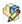in the Solidworks Assembly tab
3. In Solidworks menu click Insert/Molds/Cavity
4. In the cavity feature manager, select Charged sphere and Cavity as the Design Components
5. Click OK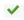.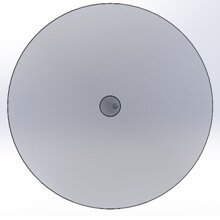Figure 4 -
3D model of sphere with a spherical cavity together with surrounding air domain

The simulation is performed as the EMS Electrostatic study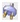. Air is used as a material for all parts.
(To see how to assign materials, see the “Computing capacitance of a multi-material capacitor” example).

### Boundary conditions

To simulate the electric field, a Charge density boundary condition should be assigned to the large sphere, and a Fixed voltage boundary condition should be assigned to the face of the Air region.

To assign a charge density to the Charged sphere

1. In the EMS manger tree, Right-click on the Load/Restraint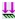, select Charge density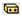, then choose Volume.
2. Click inside the Bodies Selection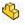box and then select the Charged sphere.
3. In the Charge Density tab, type 1e-006.
4. Click OK.

To see how to assign 0 Volt to the face of the Air region, see “Force in a capacitor” example.

## Results

To display the variation of the electric field along the axis that connects the center of the Charged sphere and the center of the cavity:

1. In the EMS manger tree, Under Results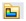, right click on the Electric Field folder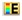and select 2D Plot then choose Linear.
2. The 2D Electric Field Property Manager Page appears.
3.  In the Select points tab, select the start and the end points.
4. In the Number of Points tab, type 1000.
5. Click OKIn the obtained curve (Figure 5), it’s clear that the electric field in the cavity is constant, and its value is, which closely matches the theoretical result. The field in Figure 5 steadily increases with the radius until it meets the cavityand then remains unchanged through the cavity (till). The field peaks at the surface of the sphere () and then it drops with the square of radius.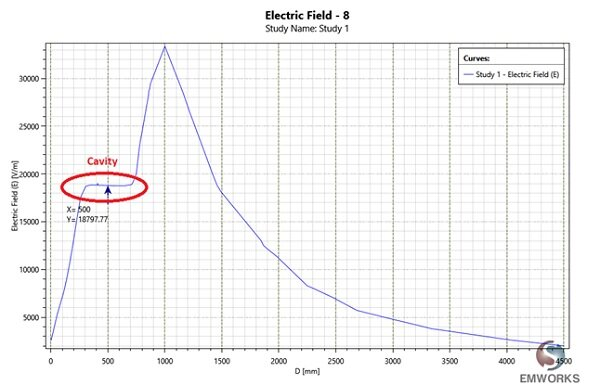Figure 5 - Electric field vs radius